# Physics Assignment Help With Work## Work Assignment Help

The work W done by a constant force F when its point of application undergoes a displacement s is defined to be
W = F s cos q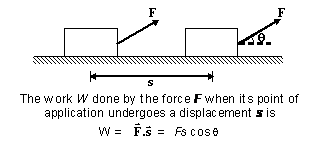where qis the angle between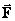and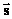as indicated in figure. Only the component ofalong s, that is, F cos q, contributes to the work done. Strictly speaking, the work is done by the source or agent that applies the force. Work is a scalar quantity and its SI unit is the joule (J).

Work is also defined as the dot product of force and its displacement as given by equation
W =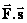In terms of rectangular components, the two vectors are= Fx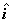+ Fy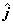+ Fz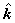and= Dx+ Dy+ Dz;

hence, the definition of work may be written as
W = Fx Dx + Fy Dy + Fz Dz

The work done by a given force on a body depends only on the force, the displacement, and the angle between them. It does not depend on the velocity or the acceleration of the body, or on the presence of other forces.

Since the work is a scalar, its value also does not depend on the orientation of the coordinate axes. Since the magnitude of a displacement in a given time interval depends on the velocity of the frame of reference used to measure the displacement, the calculated work also depends on the reference frame.

### Assignment Help For Work

assignmenthelp.net provides the best Online Assignment Help service in Physics for all standards. Our Tutor provide their high quality and optimized Tutorial help to fulfill all kind of need of Students.